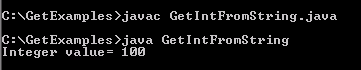Tutorials

# Java get Int from String

In this section, you will study how to obtain the integer value from String. For this, we have defined a string. The method Integer.parseInt(st) converts the string into the integer.

In this section, you will study how to obtain the integer value from String. For this, we have defined a string. The method Integer.parseInt(st) converts the string into the integer.

# Java get Int from String

In this section, you will study how to obtain the integer value from String. For this, we have defined a string. The method  Integer.parseInt(st) converts the string into the integer.

Here is the code of GetIntFromString.java

 ``` public class GetIntFromString {  public static void main(String[] args) {   String st = "100";   int i = Integer.parseInt(st);   System.out.println("Integer value= "+i);   } }```

Output will be displayed as